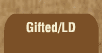Mathematics and Dyslexia

Tips for Learning the Times Tables

Tools for the Times Tables & Workbook

Home > Math > times tables practice > Eights (all)

## The Eights Times Tables - all of them

All these numbers will be even.

1. 8 x 7=
2. 8 x 1=
3. 8 x 3=
4. 8 x 6=
5. 5 x 8=
6. 0 x 8=
7. 8 x 2=
8. 4 x 8=
9. 7 x 8=
10. 9 x 8=
11. 3 x 8=
12. 6 x 8=
13. 8 x 9=
14. 8 x 10=
15. 8 x 8=